Surface Area and Volume : Exercise - 13.2 (Mathematics NCERT Class 10th)

Unless stated otherwise, take $\pi = {{22} \over 7}$.

Q.1      A solid  is in the  shape  of a cone standing on a hemisphere with both  their  radii being  equal to 1 cm and the  height  of the cone  is equal to its radius. Find the volume  of the solid in terms of $\pi$.
Sol.

Volume of the  solid = Volume  of the cone  + Volume  of the  hemisphere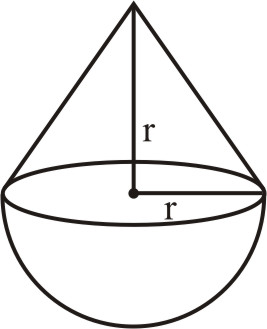$= {1 \over 3}\pi {r^2}h + {2 \over 3}\pi {R^3}$
$= {1 \over 3}\pi {r^2} \times r + {2 \over 3}\pi {r^3}$        [Since h = r and R = r]
$= {\pi \over 3}{r^3}\left( {1 + 2} \right) = {\pi z\over 3}{r^3} \times 3$
$= \pi {r^3} = \pi {\left( 1 \right)^3} = \pi \times 1 = \pi \,c{m^3}$    [Since r = 1 cm]

Q.2     Rachel an engineering student was asked to make a model shaped like a cylinder with two cones attached at its two ends by using thin aluminium sheet. The diameter of the model is 3 cm and its length is 12 cm. If each cone has a height of 2 cm,find the volume of air contained in the model the Rachel made. (Assume the outer and inner dimensions of the model be nearly the same).
Sol.

Volume  of the air  contained in the model =  Volume of the  cylindrical portion of the model + Volume  of its two  conical ends.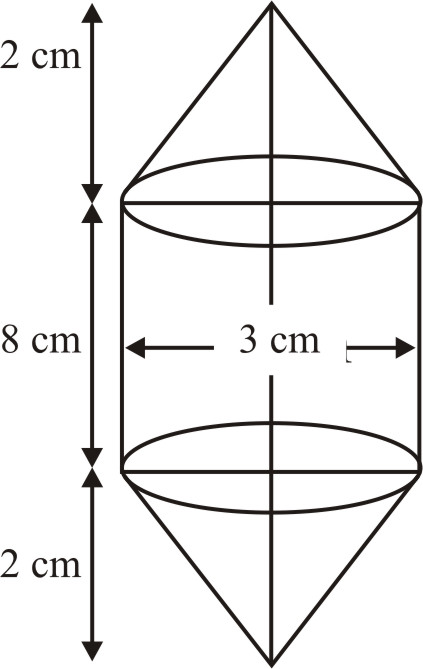$= \pi {r^2}{h_1} + 2 \times {1 \over 3}\pi {r^2}{h_2}$
$= \pi {r^2}\left( {{h_1} + {2 \over 3}{h_2}} \right)$
where $r = {3 \over 2}cm$
${h_1} = 8\,cm\,and\,\,{h_2} = 2cm$
$= {{22} \over 7} \times {\left( {{3 \over 2}} \right)^2} \times \left( {8 + {2 \over 3} \times 2} \right)c{m^3}$
$= {{22} \over 7} \times {9 \over 4} \times {{24 + 4} \over 3}c{m^3}$
$= \left( {{{22} \over 7} \times {9 \over 4} \times {{28} \over 3}} \right)c{m^3}$
$= 66\,c{m^3}\left( {approx} \right)$

Q.3       A gulab jamun, contains sugar syrup up to  about 30% of its volume. Find approximately how much  syrup would be found in 45 gulab jamuns,  each shaped like a cylinder with two hemsipherical ends with length 5 cm and diameter is 2.8 cm (see figure).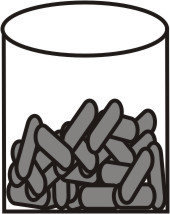Sol.

Volume of the gulab jamun = Volume of the cylindrical portion + Volume of the hemispherical ends
$= \pi {r^2}h + 2\left( {{2 \over 3}\pi {r^3}} \right)$
$= \pi {r^2}\left( {h + {4 \over 3}r} \right)$, where  r  = 1.4 cm, h = 2.2 cm
$= {{22} \over 7} \times {\left( {1.4} \right)^2} \times \left( {2.2 + {4 \over 3} \times 1.4} \right)c{m^3}$
$= {{22} \over 7} \times 1.96 \times \left( {{{6.6 + 5.6} \over 3}} \right)c{m^3}$
$= {{22} \over 7} \times 1.96 \times {{12.2} \over 3}c{m^3}$
Volume  of 45 gulab jamuns
$= 45 \times {{22} \over 7} \times 1.96 \times {{12.2} \over 3}c{m^3}$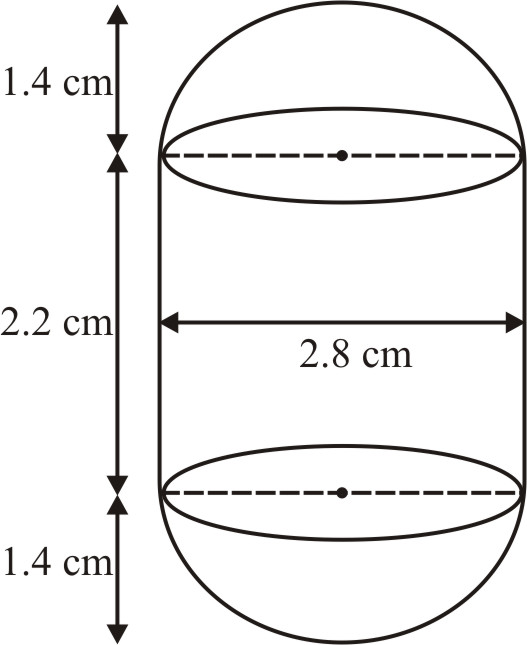Quantity of syrup in gulab jamuns  = 30% of their  volume
$= {{30} \over {100}} \times 45 \times {{22} \over 7} \times 1.96 \times {{12.2} \over 3}c{m^3}$
${{9 \times 11 \times 1.96 \times 12.2} \over 7}c{m^3} = 338.184\,c{m^3}$
$= 338\,\,c{m^3}\left( {approx} \right)$

Q.4      A pen  stand  made of wood  is in the  shape  of a cuboid  with four  conical  depressions to hold pens. The  dimensions of the cuboid are 15 cm by 10 cm by 3.5 cm. The radius of each of the depressions is 0.5 m and the  depth is 1.4 cm. Find the volume  of wood  in the  entire  stand (see figure).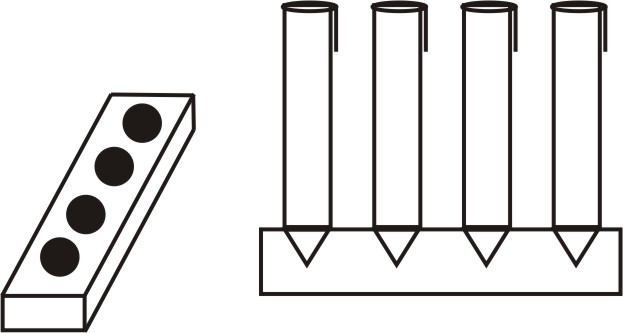Sol.

Volume  of wood  in the entire  stand   = Volume  of the cuboid – 4 × Volume  of a depression (i.e., cone)
$= 15 \times 10 \times 3.5\,c{m^3} - 4 \times {1 \over 3} \times {{22} \over 7} \times 0.5 \times 0.5 \times 1.4\,c{m^3}$
$= \left( {525 - 1.47} \right)c{m^3} = 523.53\,c{m^3}$

Q.5       A vessel in the form of an inverted cone. Its height is 8 cm and the radius of its top,  which is open, is 5 cm. It is filled with water up to the brim. When lead shots, each  of which is a sphere of radius 0.5 cm are dropped into  the vessel, one- fourth of the  water flows out. Find the  number of lead shots dropped in the vessel.
Sol.

Height  of the conical  vessel, h = 8 cm.
Its  radius r = 5 cm
Volume of cone = Volume of water in cone
$= {1 \over 3}\pi {r^2}h$
$= {1 \over 3} \times {{22} \over 7} \times 5 \times 5 \times 8\,c{m^3}$
$= {{4400} \over {21}}c{m^3}$
Volume  of water flows out = Volume of  lead shots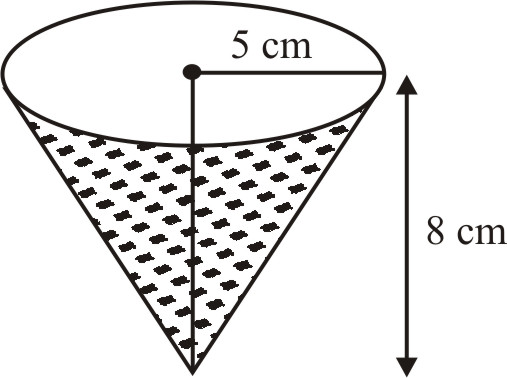$= {1 \over 4}$ of the  volume of water in the cone
$= {1 \over 4} \times {{4400} \over {21}}c{m^3} = {{1100} \over {21}}c{m^3}$
Volume of one  spherical  lead shot $= {4 \over 3}\pi {r^3}$
$= {4 \over 3} \times {{22} \over 7} \times {5 \over {10}} \times {5 \over {10}} \times {5 \over {10}}c{m^3} = {{11} \over {21}}c{m^3}$
Therefore   Number of lead  shots dropped into the vessel
$= {{Volume\,of\,water\,flows\,out} \over {Volume\,of\,one\,lead\,shot}}$
$= {{1100} \over {21}} \div {{11} \over {21}} = {{1100} \over {21}} \times {{21} \over {11}} = 100$

Q.6       A solid iron  pole  consists of a cylindrical height  220 cm and base diameter 24 cm, which  is surmounted by another cylinder of height 60 cm and radius 8 cm. Find the mass of the pole, given that 1 $c{m^3}$ of iron  has approximately 8 g mass. (Use $\pi = 3.14$)
Sol.

Volume  of the solid iron  pole = Volume  of the cylindrical  portion + Volume  of the other  cylindrical portion
$= \pi {r_1}^2{h_1} + \pi {r_2}^2{h_2}$
$= {\left( {3.14 \times (12} \right)^2} \times 220 + 3.14 \times {(8)^2} \times 60)\,c{m^3}$
$= \left( {3.14 \times 144 \times 220 + 3.14 \times 64 \times 60} \right)c{m^3}$
$= \left( {99475.2 + 12057.6} \right)c{m^3}$
$= 111532.8\,c{m^3}$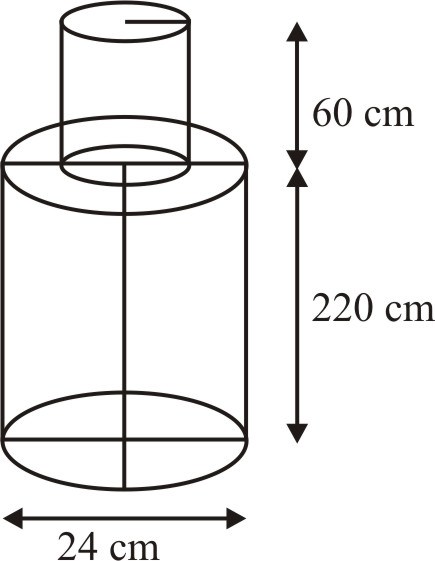Hence, the mass of the pole = (111532.8 × 8) grams
$= \left( {{{111532.8 \times 8} \over {1000}}} \right)kg = 892.26\,\,kg$

Q.7      A solid  consisting  of a right  circular  cone of height  120 cm and radius 60 cm standing on a hemisphere of radius 60 cm is placed upright in a right circular cylinder full of water circular such that it touches the bottom. Find the volume of water left in the cylinder, if the  radius of the  cylinder is 60 cm and its height is 180 cm.
Sol.

Volume  of the cylinder $= \pi {r^2}h$
$= {{22} \over 7} \times {\left( {60} \right)^2} \times 180\,c{m^3}$
$= {{22 \times 3600 \times 180} \over 7}c{m^3}$
$= {{14256000} \over 7}c{m^3}$
Volume of the  solid = Volume of cone + Volume of hemisphere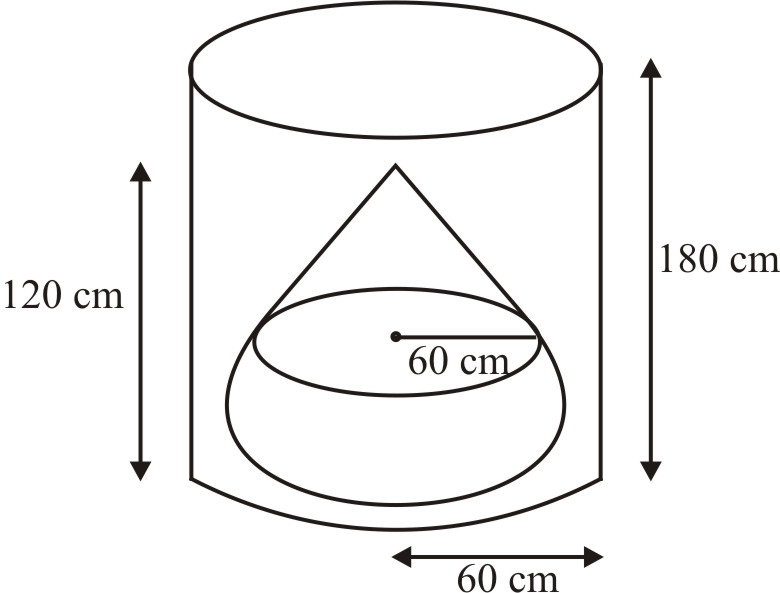$= \left( {{1 \over 3} \times {{22} \over 7} \times {{60}^2} \times 120 + {2 \over 3} \times {{22} \over 7} \times {{60}^3}} \right)c{m^3}$
$= \left( {{{3168000} \over 7} + {{3168000} \over 7}} \right)c{m^3}$
$= {{6336000} \over 7}c{m^3}$
Volume of water left in the cylinder = Volume  of the cylinder – Volume  of the solid
$= \left( {{{14256000} \over 7} - {{6336000} \over 7}} \right)c{m^3}$
$= \left( {{{7920000} \over 7}} \right)c{m^3}$
$= 1131428.57142\,c{m^3}$
$= 1.131\,{m^3}\,\left( {approx} \right)$

Q.8      A spherical  glass vessel has  a cylindrical neck 8 cm long, 2 cm in diameter  ; the  diameter of the spherical  part is 8.5 cm. By measuring the amount of water it holds , a child finds its volume to be 345 $c{m^3}$. Check whether she is correct, taking the  above as the inside measurements and $\pi = 3.14$
Sol.

Volume of spherical  vessel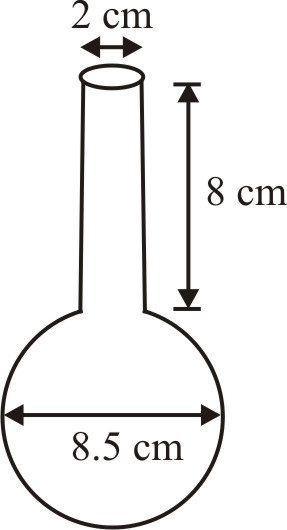$= \pi {\left( 1 \right)^2}\left( 8 \right) + {4 \over 3}\pi {\left( {4.25} \right)^3}$
$= 3.14\left[ {8 + {4 \over 3} \times 76.765625} \right]$
= 3.14[8 + 102.353]
= 3.14 × 110.353  = 346.51
Correct answer is $346.51\,c{m^3}$